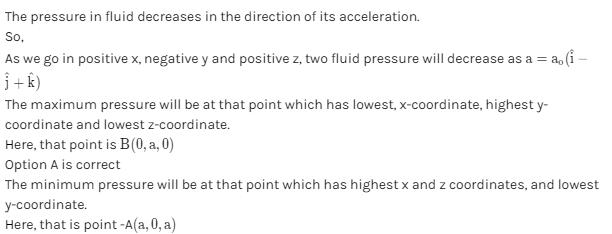Courses

# Fluid Dynamics MCQ Level - 1

## 10 Questions MCQ Test Basic Physics for IIT JAM | Fluid Dynamics MCQ Level - 1

Description
This mock test of Fluid Dynamics MCQ Level - 1 for Physics helps you for every Physics entrance exam. This contains 10 Multiple Choice Questions for Physics Fluid Dynamics MCQ Level - 1 (mcq) to study with solutions a complete question bank. The solved questions answers in this Fluid Dynamics MCQ Level - 1 quiz give you a good mix of easy questions and tough questions. Physics students definitely take this Fluid Dynamics MCQ Level - 1 exercise for a better result in the exam. You can find other Fluid Dynamics MCQ Level - 1 extra questions, long questions & short questions for Physics on EduRev as well by searching above.
QUESTION: 1

### A horizontal pipe carries water in the positive direction of x increase in the positive x-direction. Small identical holes are made at three different places along the pipe such that stream from them come out vertically upwards. The height upto which the water stream rise from the three holes :

Solution:

From Bernoulli’s theorem, water coming out from all the holes has same speed. So, they will rise to the some height.

The correct answer is:  are the same in all

QUESTION: 2

### In the following figure as shown the flow of liquid through a horizontal pipe. Three tubes A, B and C are connected to the pipe. The radii of the tubes A, B and C at the junction are respectively 2 cm, 1 cm and 2 cm. It can be said that the :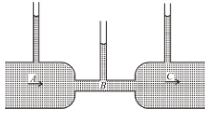Solution:

As cross-section areas of both the tubes A and C are same and tube is horizontal. Hence according to equation of continuity vA = vC and therefore according to Bernoulli’s theorem PA = PC i.e. height of liquid is same in both the tube A and C.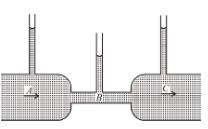The correct answer is:  Height of the liquid in the tubes A and C is the same

QUESTION: 3

### Figure shows an ideal fluid flowing through a uniform cross-sectional tube in the vertical tube with liquid velocities vA and vB  and PA and PB. Knowing that tube offers no resistance to fluid flow then which of the following is true.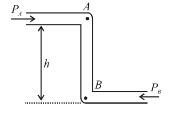Solution: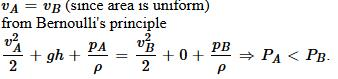QUESTION: 4

Figure shows a closed container completely filled with an ideal liquid of density ρ. In the liquid there is a spherical body of volume V and density σ attached to a string whose other end is, attached to the roof the container. The container is accelerating with an acceleration a  towards right. The force exerted by the liquid on the spherical body when it is in equilibrium with respect to the liquid will be :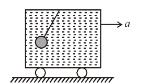Solution:

From the frame of reference of liquid, effective gravity (resultant of weight and pseudo force per unit mass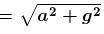)
∴ Effective force due to liquid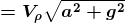Alter :

The force exerted on spherical body by surrounding liquid is equal to sum of contact force on displaced liquid by the surrounding liquid as shown in.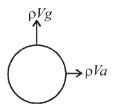Hence the force exerted in spherical body by surrounding liquid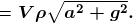The correct answer is: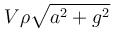QUESTION: 5

The leakage begins in water tank at position P as shown in the figure. The initial gauge pressure (pressure above that of the atmosphere) at P was 5 × 105 N/m2. If the density of water is 1000 kg/m2 the velocity with which water gushes out is :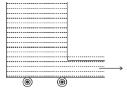Solution: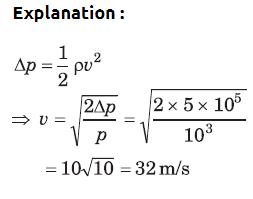QUESTION: 6

A jet of water having velocity = 10 m/s and stream cross-section = 2 cm2 hits a flat plate perpendicularly, with the water splashing out parallel to plate. The plate experiences a force of :

Solution:

The correct answer is:  20 N

QUESTION: 7

When a hole is made in the side of a container holding water, water flow out and follows a parabolic trajectory. If a hole is made in the side of the container and the container is dropped in free fall (just before the water starts coming out), the water flow (Neglect effect of the surface tension)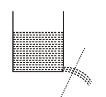Solution:

When the container is at rest with respect to the Earth, there is pressure on the walls due to the weight of the water. The pressure results from the contact force between the water and the container. In free fall, both the water and the container have acceleration of g, and the contact force is zero, so removing part of a wall by making a hole produces no outward flow. (Note that some of the water is in contact with the air, which is not accelerating, so there is still atmospheric pressure on the water.)

QUESTION: 8

The volume of a liquid flowing per second out of an orifice at the bottom of a tank does not depend upon :

Solution:

The correct answer is:  the density of the liquid

QUESTION: 9

Pressure gradient in the horizontal direction in a static fluid is represented by (z-direction to vertically upwards, and x-axis is along horizontal, d  is density of fluid) :

Solution:

In a static fluid, pressure remains same at the same level. i.e. pressure do not vary with x-coordinate.

The correct answer is: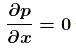*Multiple options can be correct
QUESTION: 10

The cubical container ABCDEFGH which is completely filled with an ideal (non-viscous and incompressible) fluid, moves in a gravity free space with a acceleration of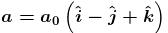where a0 is a positive constant. Then the minimum pressure at the point will be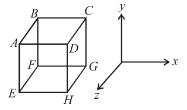Solution: